# Problem 58478. Optimal saving in Solow's classical growth model

Let us consider a simplified version of Solow's classical growth model. Let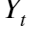,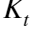,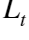,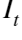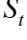and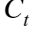denote production, the capital stock, labor, (gross) investment, savings and consumption at time t respectively (all variables are in real rather than nominal terms), and assume that output is produced using a neoclassical production function using capital and labor as inputs,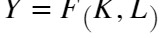, satisfying the following conditions:
1. The marginal product of capital and labor is positive:, and.
2. The marginal product of capital and labor is diminishing:, and.
3. Production exhibits constant returns to scale: F is homogenous of degree one, i.e.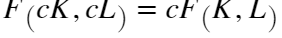for all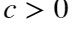.
4. F satisfies the Inada conditions: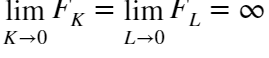, and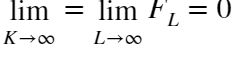.
Capital in the economy accumulates according to the law of motion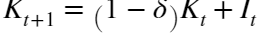, where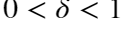is the rate of depreciation; investment equals savings, which are assumed to be a constant fraction of output,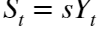for all t, for some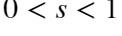. Output that is not saved is consumed (in other words, we assume a closed economy with no government activity), so that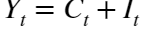for all t.
Assume that the population and hence the labor force is constant (this kind of defeats the purpose of a growth model, but we are considering a simplified version only). It is helpful to recast the model in per-capita (technically, per-laborer) terms by dividing bythroughout and taking advantage of the fact that F is homogenous of degree one. We use lower-case letters for per-capita terms:is the capital intensity,is output per capita, and so on. We also write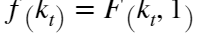; f is the intensive form of the production function F.
The model economy is in its steady state when the per-capita variables do not change; denote the steady-state capital intensity by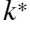. An expression implicitly characterizingcan be derived from the law of motion for capital by moving to per-capita variables and replacing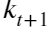and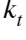withthroughout.
Since in the steady state,is constant, so is output per capita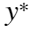and hence consumption per capita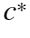.depends on three things: the depreciation rate δ, the savings rate s, and the macroeconomic production function F (equivalently, f). A social planner seeking to maximize steady-state per-capita consumption may not be able to change δ or F, but can maximizeby influencing s. We will call the savings rate that maximizes per-capita consumption the golden rule savings rate and denote it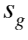; similarly, we will denote steady-state values for k, c etc. implied by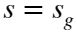as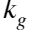,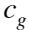and so forth.
To find, we proceed as follows:
1. find an expression forby using the relationship, moving to per-capita terms, and using the expression characterizingto replace the term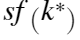with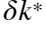;
2. take the derivative w.r.t. s, keeping in mind thatdepends on s;
3. set the resulting expression to zero, obtaining an equality identifying δ with the marginal product of capital, in per-capita terms, when the economy follows the golden rule;
4. substitute this expression back into the expression characterizingand solving for s.can then be found by again considering the relationshipin per-capita terms in the steady state, with.
Your task is now simple (in principle): assume that macroeconomic production follows a Cobb-Douglas relationship,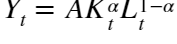,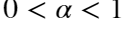(you may verify that this satisfies the conditions listed above). For given values of the (constant) technology parameter A, the capital elasticity of output α and the depreciation rate δ, please compute the golden rule savings rate, and the resulting steady-state capital intensity, per-capita output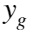and per-capita consumption.

### Solution Stats

100.0% Correct | 0.0% Incorrect
Last Solution submitted on Jul 03, 2023

### Community Treasure Hunt

Find the treasures in MATLAB Central and discover how the community can help you!

Start Hunting!